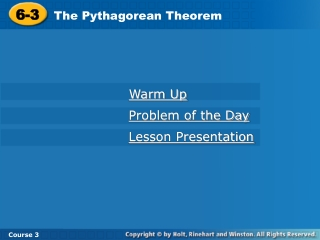# 6-3 - PowerPoint PPT PresentationDownload Presentation6-3

6-3
Download Presentation## 6-3

- - - - - - - - - - - - - - - - - - - - - - - - - - - E N D - - - - - - - - - - - - - - - - - - - - - - - - - - -
##### Presentation Transcript

1. 6-3 The Pythagorean Theorem Warm Up Problem of the Day Lesson Presentation Course 3

2. 6-3 The Pythagorean Theorem Course 3 Warm Up Graph the figures with the given verticals and find the area. 1. (–1, –1), (–1, 3), (6, –1) 2. (2, 1), (8, 1), (6, –3) 3. (3, –2), (15, –2), (14, 6), (4, 6) 14 units2 12 units2 88 units2

3. 6-3 The Pythagorean Theorem Course 3 Problem of the Day A side of a square A is 5 times the length of square B. How many times as great is the area of square A than the area of square B? 25

4. 6-3 The Pythagorean Theorem Learn to use the Pythagorean Theorem and its converse to solve problems. Course 3

5. 6-3 The Pythagorean Theorem Course 3 Vocabulary Pythagorean Theorem leg hypotenuse

6. 6-3 The Pythagorean Theorem a2 + b2 = c2 Course 3

7. 6-3 The Pythagorean Theorem 41 = c Solve for c; c = c2. Course 3 Additional Example 1A: Find the the Length of a Hypotenuse Find the length of the hypotenuse. c A. 4 5 Pythagorean Theorem a2 + b2 = c2 42 + 52 = c2 Substitute for a and b. Simplify powers. 16 + 25 = c2 41 = c2 6.40c

8. 6-3 The Pythagorean Theorem Solve for c; c = c2. 225 = c Course 3 Additional Example 1B: Find the the Length of a Hypotenuse Find the length of the hypotenuse. triangle with coordinates B. (1, –2), (1, 7), and (13, –2) Pythagorean Theorem a2 + b2 = c2 Substitute for a and b. 92 + 122 = c2 Simplify powers. 81 + 144 = c2 15= c

9. 6-3 The Pythagorean Theorem 74 = c Solve for c; c = c2. Course 3 Try This: Example 1A Find the length of the hypotenuse. c A. 5 7 Pythagorean Theorem a2 + b2 = c2 52 + 72 = c2 Substitute for a and b. Simplify powers. 25 + 49 = c2 8.60c

10. 6-3 The Pythagorean Theorem y x Solve for c; c = c2. 61 = c Course 3 Try This: Example 1B Find the length of the hypotenuse. B. triangle with coordinates (–2, –2), (–2, 4), and (3, –2) (–2, 4) The points form a right triangle. a2 + b2 = c2 Pythagorean Theorem 62 + 52 = c2 Substitute for a and b. 36 + 25 = c2 Simplify powers. (3, –2) (–2, –2) 7.81c

11. 6-3 The Pythagorean Theorem 576 = 24 Course 3 Additional Example: 2 Finding the Length of a Leg in a Right Triangle Solve for the unknown side in the right triangle. Pythagorean Theorem a2 + b2 = c2 25 Substitute for a and c. 72 + b2 = 252 b Simplify powers. 49 + b2 = 625 –49 –49 b2 = 576 7 b = 24

12. 6-3 The Pythagorean Theorem 128  11.31 Course 3 Try This: Example 2 Solve for the unknown side in the right triangle. a2 + b2 = c2 Pythagorean Theorem 12 Substitute for a and c. b 42 + b2 = 122 Simplify powers. 16 + b2 = 144 –16 –16 4 b2 = 128 b 11.31

13. 6-3 The Pythagorean Theorem a = 20 units ≈ 4.47 units 1 2 1 2 A = hb = (8)( 20) = 4 20 units2 17.89 units2 Course 3 Additional Example 3: Using the Pythagorean Theorem to Find Area Use the Pythagorean Theorem to find the height of the triangle. Then use the height to find the area of the triangle. a2 + b2 = c2 Pythagorean Theorem Substitute for b and c. a2 + 42 = 62 a2 + 16 = 36 6 6 a a2 = 20 4 4 Find the square root of both sides.

14. 6-3 The Pythagorean Theorem a = 21 units ≈ 4.58 units 1 2 1 2 A = hb = (4)( 21) = 2 21 units2 4.58 units2 Course 3 Try This: Example 3 Use the Pythagorean Theorem to find the height of the triangle. Then use the height to find the area of the triangle. a2 + b2 = c2 Pythagorean Theorem a2 + 22 = 52 Substitute for b and c. 5 5 a2 + 4 = 25 a a2 = 21 2 2 Find the square root of both sides.

15. 6-3 The Pythagorean Theorem Course 3 Lesson Quiz Use the figure for Problems 1-3. 1. Find the height of the triangle. 8m 2. Find the length of side c to the nearest meter. c 10 m h 12m 3. Find the area of the largest triangle. 6 m 9 m 60m2 4. One leg of a right triangle is 48 units long, and the hypotenuse is 50 units long. How long is the other leg? 14 units